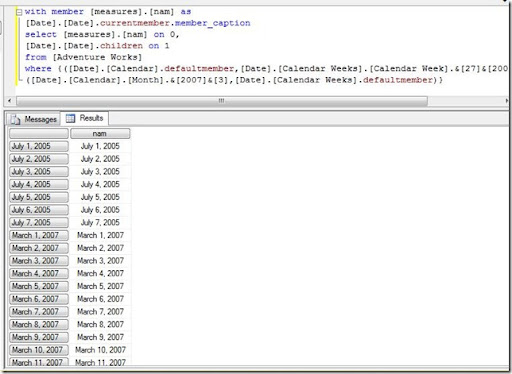# OR operation in MDX

A seemingly harmless question which makes you ignore it thinking you know the answer, but the moment you give it a second look, you start scratching your head. This was my first reaction when I was asked to implement an OR operation in MDX and I really had to think to come up with an answer, quite contrary to the no-brainer I thought this question was. Ever since, this has been in my list of interview questions and this blog would be the second part of the series where I unveil some of my favourite questions. Problem Lets face a scenario where you have a relation – Month<—Date & Week<—Date. Now the requirement is such that the Date field should be displayed such that it should either belong to the Month selected or the Week selected. Answer Follow the steps below to get the answer 1) The query below will display the Date member captions for all dates WITH
MEMBER [measures].[nam] AS
[Date].[Date].CurrentMember.Member_Caption
SELECT
[measures].[nam] ON 0
,[Date].[Date].Children ON 1
FROM [Adventure Works]; 2) Suppose we need to display all the Dates such that it either belongs to the month March,2007 or Week 27 CY 2005. P.S. : Just giving a where clause with a set like the query given below will not work as it will perform an AND operation rather than an OR operation. WITH
MEMBER [measures].[nam] AS
[Date].[Date].CurrentMember.Member_Caption
SELECT
[measures].[nam] ON 0
,[Date].[Date].Children ON 1
WHERE
(
{[Date].[Calendar Weeks].[Calendar Week].&&}
,{[Date].[Calendar].[Month].&&}
); 3) The correct way is to bring both the conditions inside a set but as of now, they are of different dimensionality and can’t be used together as the members of the same set. Hence we create a tuple of the calendar week and month such that the valid condition of one attribute would be used with the default member of the other dimension (which is usually the All member). WITH
MEMBER [measures].[nam] AS
[Date].[Date].CurrentMember.Member_Caption
SELECT
[measures].[nam] ON 0
,[Date].[Date].Children ON 1
WHERE
{
(
[Date].[Calendar].DefaultMember
,[Date].[Calendar Weeks].[Calendar Week].&&
)
,(
[Date].[Calendar].[Month].&&
,[Date].[Calendar Weeks].DefaultMember
)
}; This query would give the correct output as shown belowThis query makes use of the fact that ([Date].[Calendar].DefaultMember,[Date].[Calendar Weeks].[Calendar Week].&&) = (Date].[Calendar Weeks].[Calendar Week].&&) We use this technique to make the dimensionality of both the week and month conditions the same. Once this concept is understood, implementing OR conditions in MDX would be a piece of cake.### Posted by SQLJason

This site uses Akismet to reduce spam. Learn how your comment data is processed.